• 多段图，求最短路径，如： 对其使用动态规划法： 阶段：将中的顶点划分5个阶段，k 状态：每个阶段有几种供选择的点s 决策：当前状态应在前一个状态的基础上获得。决策需要满足规划方程 规划方程：f(k)...
对多段图，求最短路径，如图：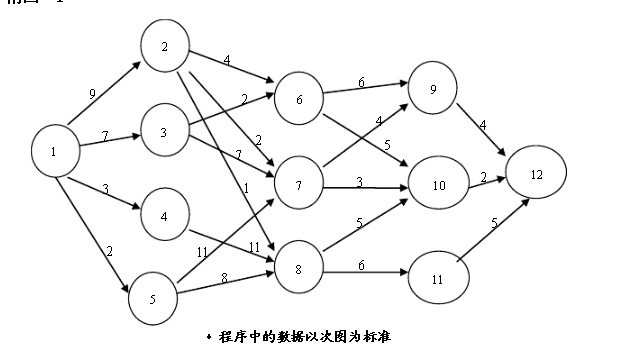对其使用动态规划法：
阶段：将图中的顶点划分5个阶段，k
状态：每个阶段有几种供选择的点s
决策：当前状态应在前一个状态的基础上获得。决策需要满足规划方程
规划方程：f(k)表示状态k到终点状态的最短距离。
初始条件：f(k)=0;
方程：f(k-1)=min{f(k)+W(k-1,k)}其中W(k-1,k)表示状态k-1到状态k的距离
代码如下：
#include<limits.h>
#include<iostream>
using namespace std;
void Init_Graph(int N,int k,int** S, int **C)
{
int X;
int i,j;
int temp=N;
cout<<"输入边的长度：输入1 2 4 表示点1 与 2的边的长度为 4：首数字为0表示结束输入"<<endl;
cin>>i;
while (i!=0)
{
cin>>j;
cin>>C[i][j];
cin>>i;
}
cout<<"输入每个阶段有哪些点：输入：X 1 2 3表示该阶段有X个点，分别为1,2,3："<<endl;
for (i=1;i<=k;i++)
{
cout<<"输入第"<<i<<"阶段的状态的点数：";
cin>>X;
cout<<"这些点分别为：";
for (j=0;j<X;j++)
{
cin>>S[i][j];
}
}
}
void Plan(int N,int k,int **S,int **F,int** C,int *result)
{
int i,j,t=N;
int m;
int point;
//cout<<S[k]<<" ";
point=S[k];
for (i=k-1;i>=1;i--)//阶段
{
j=0;//i阶段的状态
while (S[i][j]!=0)//状态
{
m=0;//i+1阶段的状态
F[i][j]=INT_MAX;
if (C[S[i][j]][point]==INT_MAX)
{
while (S[i+1][m]!=0)
{
if (C[S[i][j]][S[i+1][m]]!=INT_MAX)
{
if (F[i][j]>(F[i+1][m]+C[S[i][j]][S[i+1][m]]))
{
F[i][j] = F[i+1][m] + C[S[i][j]][S[i+1][m]];
result[S[i][j]]=S[i+1][m];
t--;
}
}
m++;
}
}
else
{
while (S[i+1][m]!=0)
{
if (F[i][j]>(F[i+1][m]+C[S[i][j]][S[i+1][m]]))
{
F[i][j] = F[i+1][m] + C[S[i][j]][S[i+1][m]];
result[S[i][j]]=S[i+1][m];
t--;
}
m++;
}
}
j++;
}
}
cout<<"符合条件的点为："<<endl;
t=0;
result[t]=1;
cout<<result[t]<<" ";
t=result[t];
while (t<N)
{
cout<<result[t]<<" ";
t=result[t];
}
cout<<endl<<"最短距离为：";
cout<<F[i+1]<<endl;
}
int main(int argc,char *argv[])
{
int N,k;
int i,j;
int **C,**S,**F;//C:边的长度，S;每个阶段的状态；F：记录每个阶段的状态中的点到终点的距离
int *result;//输出点
cout<<"输入点的个数：";
cin>>N;
cout<<"输入阶段数：";
cin>>k;
C=new int*[N+1];
//C=(int **)malloc(sizeof(int*)*(N+1));
for (i=0;i<N+1;i++)
{
//C[i]=(int *)malloc(sizeof(int)*(N+1));
C[i]=new int[N+1];
for (j=0;j<N+1;j++)
{
C[i][j]=INT_MAX;
}
}
S= new int*[N+1];
for (i=0;i<N+1;i++)
{
S[i]=new int[N+1];
memset(S[i],0,sizeof(int)*(N+1));
}
F=new int *[N+1];
for (i=0;i<N+1;i++)
{
F[i]=new int [N+1];
for (j=0;j<N+1;j++)
{
F[i][j]=0;
}
}
result=new int[N+1];
memset(result,0,sizeof(int)*(k+1));
Init_Graph(N,k,S,C);
/*
多段图的动态规划方法
阶段：k
状态：Sk:即每个阶段可供选择的点的位置
决策：u
规划方程：f(k)=min(f(k+1)+边u的长度。
f(k)表示：k点到终点的最短路径长度
初始值：F(k)=0;
*/
Plan(N,k,S,F,C,result);
delete []result;
for (i=0;i<N+1;i++)
{
delete []C[i];
}
delete []C;
for (i=0;i<N+1;i++)
{
delete []S[i];
}
delete []S;
for (i=0;i<N+1;i++)
{
delete []F[i];
}
delete []F;
return 0;
}
运行结果如下：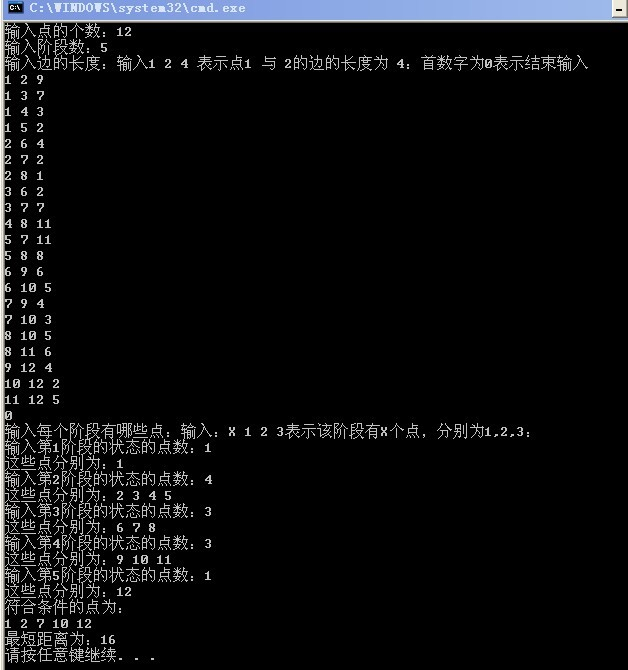展开全文• 应用示例： ...在位置与目的地，路径规划会...从起始点到达目的地的最佳行车路线的过程，它是多段图最短路径问题的典型应用。 对以下多段图，求出从原点1到终点10的最短路径。 分析： 源代码： #include <iostr...
应用示例： 在车辆中安装了车辆导航系统，人们就可以不必为迷路、绕远而担心，只需点击所 在位置与目的地，路径规划会帮你找出到达目的地的最短路线。 路径规划是基于具有拓扑结构的道路网络，在车辆行驶前或行驶过程中寻找车辆 从起始点到达目的地的最佳行车路线的过程，它是多段图最短路径问题的典型应用。 对以下多段图，求出从原点1到终点10的最短路径。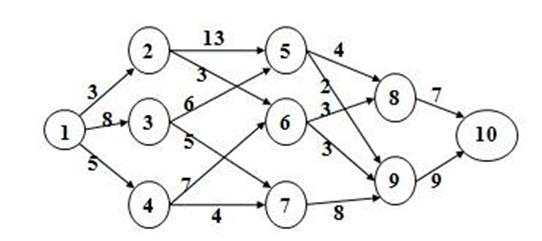分析：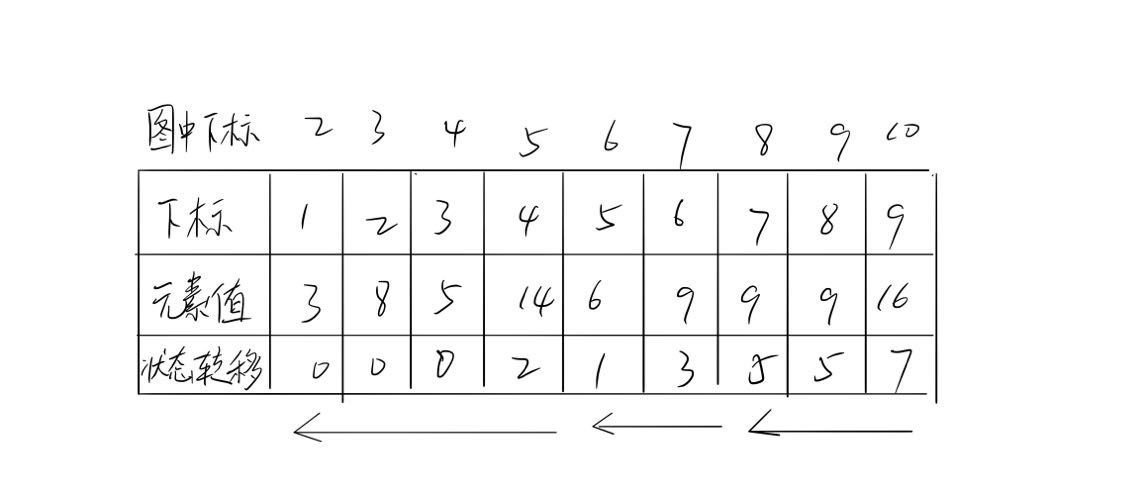第二行是cost数组，第三行是path数组
输入： 输入： 10 16 0 1 3 0 2 8 0 3 5 1 4 13 1 5 3 2 4 6 2 6 5 3 5 7 3 6 4 4 7 4 4 8 2 5 7 3 5 8 3 6 8 8 7 9 7 8 9 9
源代码：
#include <iostream>
using namespace std;
const int N = 20;
const int MAX = 1000;
int arc[N][N]; //存储弧上的权值

int CreatGraph(){
int i,j,k;
int weight;
int vnum,arcnum;
cout<<"请输入顶点个数和边的个数：";
cin>>vnum>>arcnum;
for(i=0;i<vnum;i++)
for(j=0;j<vnum;j++)
arc[i][j]=MAX;
for(k=0;k<arcnum;k++){
cout<<"请输入边的两个顶点和权值:";
cin>>i>>j>>weight;
arc[i][j]=weight;
}
return vnum;
}

int BackPath(int n){
int i,j;
int cost[N];int path[N];
for(i=1;i<n;i++)
{
cost[i]=MAX;
path[i]=-1;
}
cost=0;path=-1;
for(j=1;j<n;j++){
for(i=j-1;i>=0;i--)
{
if(arc[i][j]+cost[i]<cost[j])
{
cost[j]=arc[i][j]+cost[i];
path[j]=i;
}
}
}
cout<<"路径是倒序:";
cout<<n;
i=n-1;
while(path[i]>=0){
cout<<"<-"<<path[i]+1;
i=path[i];
}
return cost[n-1];
}

int main()
{     int n = CreatGraph( );
int pathLen = BackPath(n);
cout<<endl<<"最短路径的长度是:"<<pathLen<<endl;
return 0;
}


运行结果：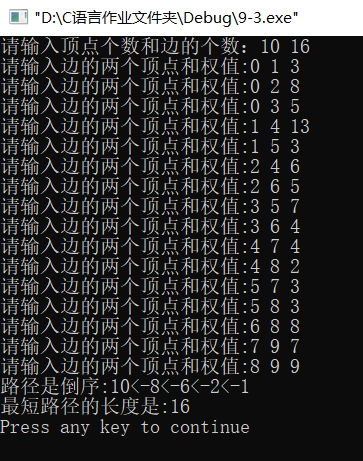时间复杂度：理论时间复杂度：O（m+k）–(m为边数个数，k为划分段数), 实际时间复杂度O(n^2)
展开全文• 中的某个顶点出发到达另外一个顶点的所经过的边的权重和最小的一条路径，称为最短路径 解决问题的算法： 迪杰斯特拉算法（Dijkstra算法） 弗洛伊德算法（Floyd算法） SPFA算法 之前已经对D...
Genius only means hard-working all one’s life.  Name:Willam  Time:2017/3/8
1、最短路径问题介绍
问题解释：  从图中的某个顶点出发到达另外一个顶点的所经过的边的权重和最小的一条路径，称为最短路径
解决问题的算法：
迪杰斯特拉算法（Dijkstra算法）弗洛伊德算法（Floyd算法）SPFA算法
之前已经对Dijkstra算法做了介绍（不懂的可以看这篇博客：Dijkstra算法详解），所以这篇博客打算对Floyd算法做详细的的介绍。
2、Floyd算法的介绍
算法的特点：  弗洛伊德算法是解决任意两点间的最短路径的一种算法，可以正确处理有向图或有向图或负权（但不可存在负权回路)的最短路径问题，同时也被用于计算有向图的传递闭包。  算法的思路
通过Floyd计算图G=(V,E)中各个顶点的最短路径时，需要引入两个矩阵，矩阵S中的元素a[i][j]表示顶点i(第i个顶点)到顶点j(第j个顶点)的距离。矩阵P中的元素b[i][j]，表示顶点i到顶点j经过了b[i][j]记录的值所表示的顶点。
假设图G中顶点个数为N，则需要对矩阵D和矩阵P进行N次更新。初始时，矩阵D中顶点a[i][j]的距离为顶点i到顶点j的权值；如果i和j不相邻，则a[i][j]=∞，矩阵P的值为顶点b[i][j]的j的值。 接下来开始，对矩阵D进行N次更新。第1次更新时，如果”a[i][j]的距离” > “a[i]+a[j]”(a[i]+a[j]表示”i与j之间经过第1个顶点的距离”)，则更新a[i][j]为”a[i]+a[j]”,更新b[i][j]=b[i]。 同理，第k次更新时，如果”a[i][j]的距离” > “a[i][k-1]+a[k-1][j]”，则更新a[i][j]为”a[i][k-1]+a[k-1][j]”,b[i][j]=b[i][k-1]。更新N次之后，操作完成！
3、Floyd算法的实例过程
上面，我们已经介绍了算法的思路，如果，你觉得还是不理解，那么通过一个实际的例子，把算法的过程过一遍，你就明白了，如下图，我们求下图的每个点对之间的最短路径的过程如下：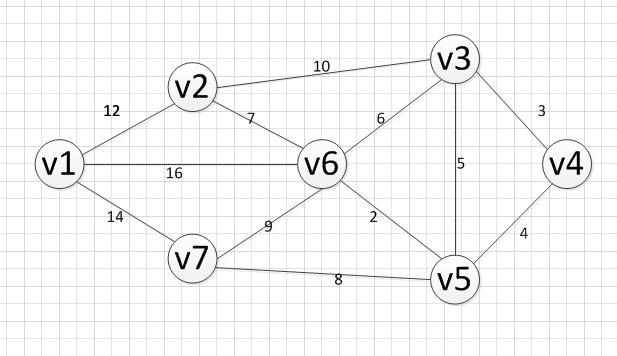第一步，我们先初始化两个矩阵，得到下图两个矩阵：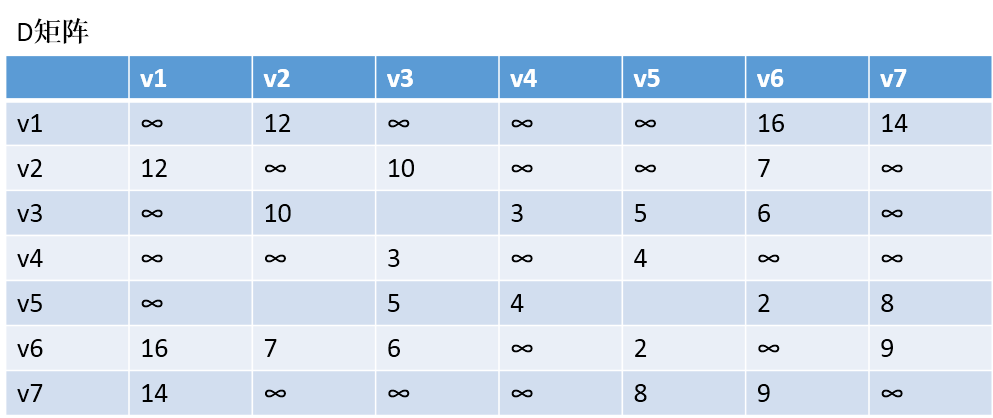、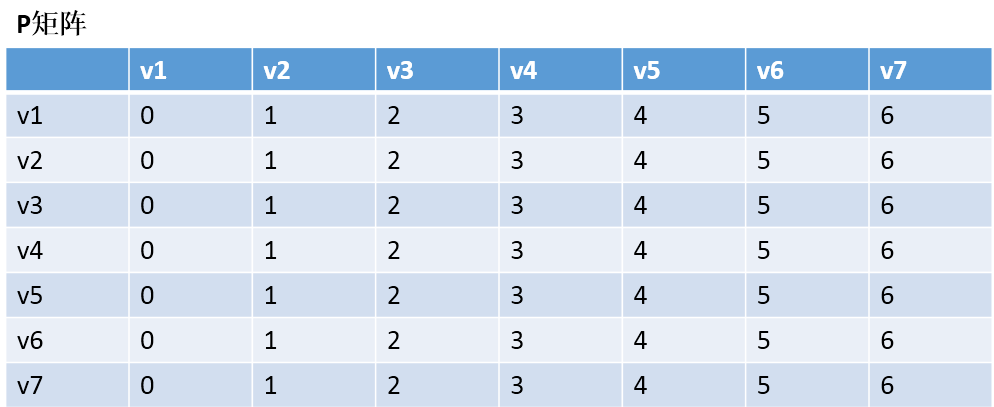第二步，以v1为中阶，更新两个矩阵：  发现，a+a < a 和a+a < a，所以我们只需要矩阵D和矩阵P，结果如下：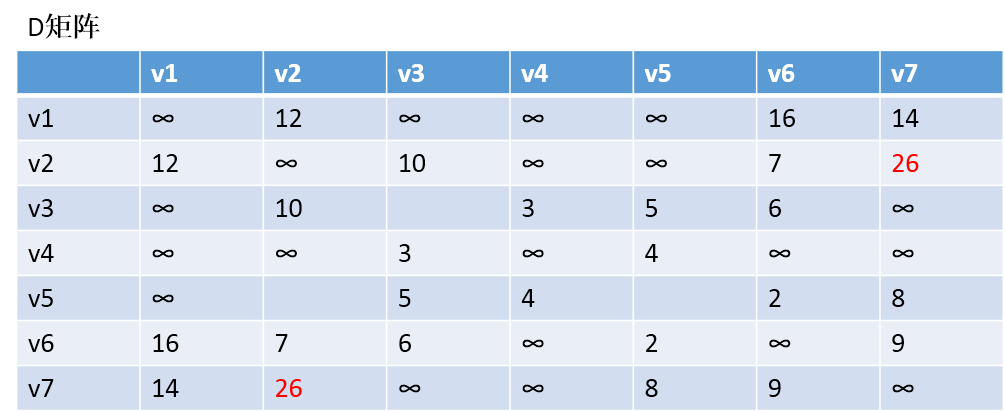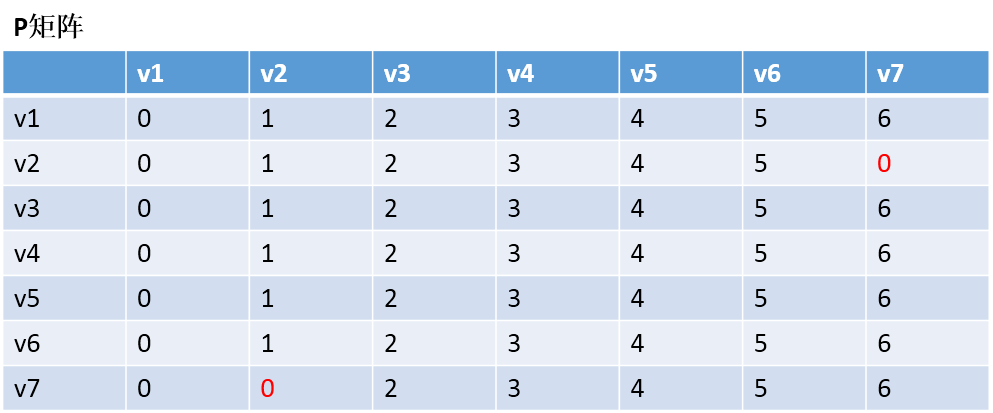通过矩阵P，我发现v2–v7的最短路径是：v2–v1–v7
第三步：以v2作为中介，来更新我们的两个矩阵，使用同样的原理，扫描整个矩阵，得到如下图的结果：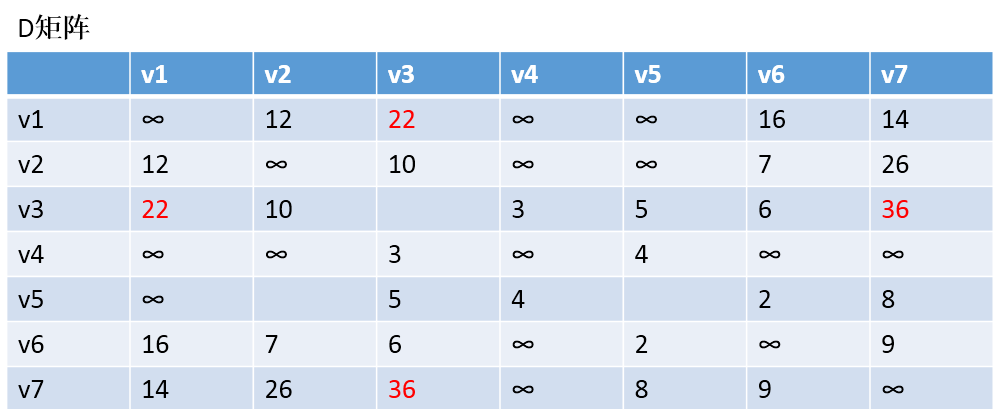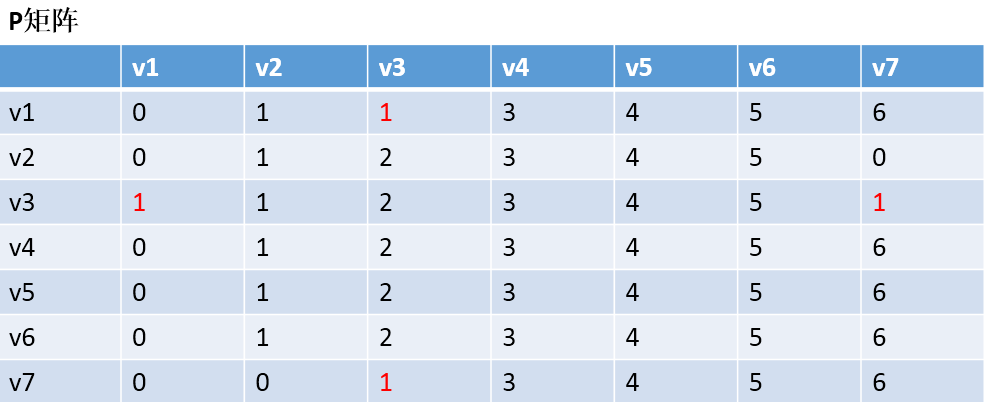OK，到这里我们也就应该明白Floyd算法是如何工作的了，他每次都会选择一个中介点，然后，遍历整个矩阵，查找需要更新的值，下面还剩下五步，就不继续演示下去了，理解了方法，我们就可以写代码了。
4、Floyd算法的代码实现
Floyd.h文件代码
/************************************************************/
/*                程序作者：Willam                          */
/*                程序完成时间：2017/3/11                   */
/*                有任何问题请联系：2930526477@qq.com       */
/************************************************************/
//@尽量写出完美的程序

#pragma once
//#pragma once是一个比较常用的C/C++杂注，
//只要在头文件的最开始加入这条杂注，
//就能够保证头文件只被编译一次。

/*
本博客开始对Floyd算法的使用邻接矩阵实现的
*/

#include<iostream>
#include<string>
using namespace std;

class Graph_DG {
private:
int vexnum;   //图的顶点个数
int edge;     //图的边数
int **arc;   //邻接矩阵
int ** dis;   //记录各个顶点最短路径的信息
int ** path;  //记录各个最短路径的信息
public:
//构造函数
Graph_DG(int vexnum, int edge);
//析构函数
~Graph_DG();
// 判断我们每次输入的的边的信息是否合法
//顶点从1开始编号
bool check_edge_value(int start, int end, int weight);
//创建图
void createGraph(int);
//打印邻接矩阵
void print();
//求最短路径
void Floyd();
//打印最短路径
void print_path();
};


Floyd.cpp文件代码
#include"Floyd.h"

//构造函数
Graph_DG::Graph_DG(int vexnum, int edge) {
//初始化顶点数和边数
this->vexnum = vexnum;
this->edge = edge;
//为邻接矩阵开辟空间和赋初值
arc = new int*[this->vexnum];
dis = new int*[this->vexnum];
path = new int*[this->vexnum];
for (int i = 0; i < this->vexnum; i++) {
arc[i] = new int[this->vexnum];
dis[i] = new int[this->vexnum];
path[i] = new int[this->vexnum];
for (int k = 0; k < this->vexnum; k++) {
//邻接矩阵初始化为无穷大
arc[i][k] = INT_MAX;
}
}
}
//析构函数
Graph_DG::~Graph_DG() {

for (int i = 0; i < this->vexnum; i++) {
delete this->arc[i];
delete this->dis[i];
delete this->path[i];

}
delete dis;
delete arc;
delete path;
}

// 判断我们每次输入的的边的信息是否合法
//顶点从1开始编号
bool Graph_DG::check_edge_value(int start, int end, int weight) {
if (start<1 || end<1 || start>vexnum || end>vexnum || weight < 0) {
return false;
}
return true;
}

void Graph_DG::createGraph(int kind) {
cout << "请输入每条边的起点和终点（顶点编号从1开始）以及其权重" << endl;
int start;
int end;
int weight;
int count = 0;
while (count != this->edge) {
cin >> start >> end >> weight;
//首先判断边的信息是否合法
while (!this->check_edge_value(start, end, weight)) {
cout << "输入的边的信息不合法，请重新输入" << endl;
cin >> start >> end >> weight;
}
//对邻接矩阵对应上的点赋值
arc[start - 1][end - 1] = weight;
//无向图添加上这行代码
if(kind==2)
arc[end - 1][start - 1] = weight;
++count;
}
}

void Graph_DG::print() {
cout << "图的邻接矩阵为：" << endl;
int count_row = 0; //打印行的标签
int count_col = 0; //打印列的标签
//开始打印
while (count_row != this->vexnum) {
count_col = 0;
while (count_col != this->vexnum) {
if (arc[count_row][count_col] == INT_MAX)
cout << "∞" << " ";
else
cout << arc[count_row][count_col] << " ";
++count_col;
}
cout << endl;
++count_row;
}
}

void Graph_DG::Floyd() {
int row = 0;
int col = 0;
for (row = 0; row < this->vexnum; row++) {
for (col = 0; col < this->vexnum; col++) {
//把矩阵D初始化为邻接矩阵的值
this->dis[row][col] = this->arc[row][col];
//矩阵P的初值则为各个边的终点顶点的下标
this->path[row][col] = col;
}
}

//三重循环，用于计算每个点对的最短路径
int temp = 0;
int select = 0;
for (temp = 0; temp < this->vexnum; temp++) {
for (row = 0; row < this->vexnum; row++) {
for (col = 0; col < this->vexnum; col++) {
//为了防止溢出，所以需要引入一个select值
select = (dis[row][temp] == INT_MAX || dis[temp][col] == INT_MAX) ? INT_MAX : (dis[row][temp] + dis[temp][col]);
if (this->dis[row][col] > select) {
//更新我们的D矩阵
this->dis[row][col] = select;
//更新我们的P矩阵
this->path[row][col] = this->path[row][temp];
}
}
}
}
}

void Graph_DG::print_path() {
cout << "各个顶点对的最短路径：" << endl;
int row = 0;
int col = 0;
int temp = 0;
for (row = 0; row < this->vexnum; row++) {
for (col = row + 1; col < this->vexnum; col++) {
cout << "v" << to_string(row + 1) << "---" << "v" << to_string(col+1) << " weight: "
<< this->dis[row][col] << " path: " << " v" << to_string(row + 1);
temp = path[row][col];
//循环输出途径的每条路径。
while (temp != col) {
cout << "-->" << "v" << to_string(temp + 1);
temp = path[temp][col];
}
cout << "-->" << "v" << to_string(col + 1) << endl;
}

cout << endl;
}
}

main.cpp文件的代码
#include"Floyd.h"

//检验输入边数和顶点数的值是否有效，可以自己推算为啥：
//顶点数和边数的关系是：((Vexnum*(Vexnum - 1)) / 2) < edge
bool check(int Vexnum, int edge) {
if (Vexnum <= 0 || edge <= 0 || ((Vexnum*(Vexnum - 1)) / 2) < edge)
return false;
return true;
}
int main() {
int vexnum; int edge;
cout << "输入图的种类：1代表有向图，2代表无向图" << endl;
int kind;
cin >> kind;
//判读输入的kind是否合法
while (1) {
if (kind == 1 || kind == 2) {
break;
}
else {
cout << "输入的图的种类编号不合法，请重新输入：1代表有向图，2代表无向图" << endl;
cin >> kind;
}
}

cout << "输入图的顶点个数和边的条数：" << endl;
cin >> vexnum >> edge;
while (!check(vexnum, edge)) {
cout << "输入的数值不合法，请重新输入" << endl;
cin >> vexnum >> edge;
}
Graph_DG graph(vexnum, edge);
graph.createGraph(kind);
graph.print();
graph.Floyd();
graph.print_path();
system("pause");
return 0;
}
输入：
2
7 12
1 2 12
1 6 16
1 7 14
2 3 10
2 6 7
3 4 3
3 5 5
3 6 6
4 5 4
5 6 2
5 7 8
6 7 9 
输出：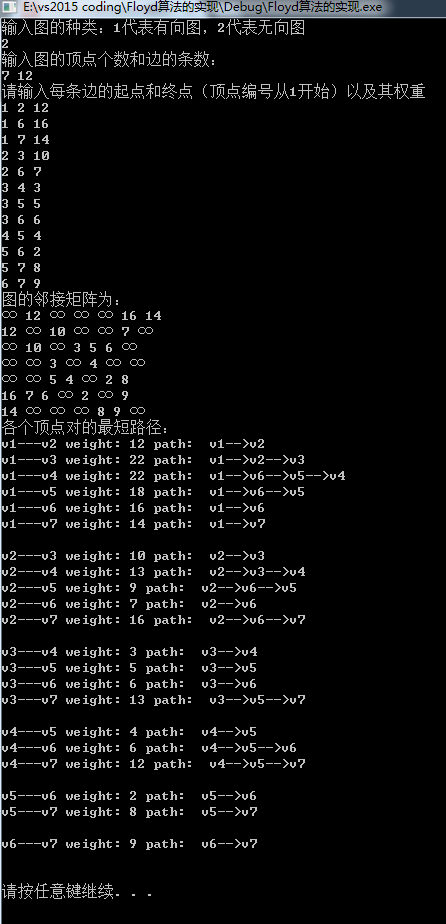展开全文• 无权最短路径 按照路径长度递增的顺序找出源点到各个顶点的最短路径 类似于BFS-宽度优先遍历，可以通过队列来实现， 先让顶点入队，循环执行下列步骤 出队首元素，访问其所有邻接点 标明源点到这些邻接点...
最短路径
–在网络中，求两个不同顶点之间的所有路径中，边的权值之和最小的路劲。
单源最短路径
–从某固定源点出发的最短路径
无权图的最短路径
按照路径长度递增的顺序找出源点到各个顶点的最短路径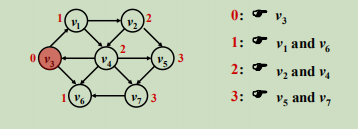类似于BFS-宽度优先遍历，可以通过队列来实现，
先让顶点入队，循环执行下列步骤出队首元素，访问其所有邻接点标明源点到这些邻接点的路径长度，并将其入队
有权图的最短路径
Dijkstra算法
令第一组为集合S={源点+已经确定最短路径的顶点vi}  令第二组为未在集合S中的顶点v，定义length成员为源点s到v的最短路径长度，该路径除了终点v以外，所有顶点都必须是集合S中的顶点。即{s—>（vi∈S）—>v}的最小长度  每次对未在第一组集合S的顶点，选一个length最小的顶点(记为v)，将其增加至集合S中。
如何选这个顶点V–用一个最小堆H(刚开始只有源点)，每次找出并删除一个length值最小的顶点V，这里这个找到并删除的顶点V就是每次加入集合S的顶点，然后找到V的所有邻接点，对其邻接点的length值进行赋值，然后加入最小堆H里，往复循环，直至最小堆空了(最小堆空了，即所有顶点都加入了集合S)–看程序就明白了  选进去的时候要注意什么–增加v进集合S中会影响顶点w(w为v的邻接点)的length值，因为多了个顶点v，可能会改变s—w的走法，原先不经过v，现在最短路径经过v，即需要考虑 length[w],与length[v] + e.weigth的大小关系--------(1)   直到把所有顶点都送进集合S中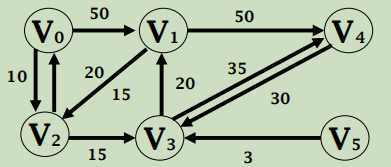设源点为V0，则按照Dijkstra算法的最短路径求解过程如下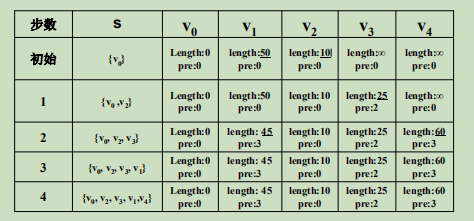注：初始的时候只有集合S只有源点s，因此只有到点v1、v2有路径，路径长度用length表示，，没有路径则length为∞，路径的终点的前一个点用pre表示。
C++
class Dist { // Dist类，用于保存最短路径信息
public:
int index; // 结点的索引值
int length; // 当前最短路径长度
int pre; // 路径最后经过的结点
};

void Dijkstra(Graph& G, int s, Dist* &D) { // s是源点
D = new Dist[G. VerticesNum()]; // 开辟空间给类Dist，用来记录当前最短路径长度

for (int i = 0; i < G.VerticesNum(); i++) { // 初始化，将图中所有顶点的标志位记为未访问，将Dist类的索引值记为顶点号，将顶点到源点的length置为∞。
G.Mark[i] = UNVISITED;
D[i].index = i;
D[i].length = INFINITE;
D[i].pre = s;
}
D[s].length = 0; // 初始化，源点自身的路径长度置为0

MinHeap<Dist> H(G. EdgesNum()); // 最小堆用于找出集合S中到源点的路径最短的点，最小堆存放Dist类元素，共有G.EdgesNum个长度
H.Insert(D[s]);  //将源点放入最小堆

for (i = 0; i < G.VerticesNum(); i++) {
bool FOUND = false;
Dist d;
while (!H.isEmpty()) {
d = H.RemoveMin(); //获得集合S中到源点s路径长度最小的结点，并删除
if (G.Mark[d.index] == UNVISITED) { //如果未访问过则跳出循环
FOUND = true; break;
}
}
if (!FOUND) break; // 若没有符合条件的最短路径则跳出本次循环
int v = d.index;
G.Mark[v] = VISITED; // 将标记位设置为 VISITED
for (Edge e = G.FirstEdge(v); G.IsEdge(e); e = G.NextEdge(e)) // 对最小堆中到源点路径最短的顶点v，求他的每个邻接点，并考虑是否需要改变其最小距离--刷新最短路，然后将其加入最小堆
if (D[G.ToVertex(e)].length > (D[v].length+G.Weight(e))) {//这里第一次执行时，因为刚开始最小堆里只有源点，其他顶点到源点的length都为∞，执行完后V1、V2的length值才改变
D[G.ToVertex(e)].length = D[v].length + G.Weight(e);
D[G.ToVertex(e)].pre = v;
H.Insert(D[G.ToVertex(e)]);
}
}
}



Dijkstra算法时间复杂度

T

=

O

(

∣

V

∣

l

o

g

∣

V

∣

+

∣

E

∣

l

o

g

∣

V

∣

)

=

O

(

∣

E

∣

l

o

g

∣

V

∣

)

T = O( |V| log|V| + |E| log|V| ) = O( |E| log|V| )

前半部分为在最小堆里找V次，依次复杂度为logV，后半部分是往最小堆里插入Dist，一次最多有可能插E次(极限情况，一个顶点有E条边)
Dijkstra使用条件
图中不能出现有总权值为负值的回路如果存在负值边也有可能发生计算错误持负权值的最短路径算法有Ford算法、SPFA算法
多源最短路径
–求每对顶点间的最短路径
Floyd算法
Dk[i] [j]表示路径{ i —> { l<= k } —> j }的最小长度（i、j、l、k表示顶点编号）  首先用矩阵D0表示该图的邻接矩阵  在矩阵D0上做n次迭代  如何迭代呢–当Dk-1已经完成，递推到Dk时，
若k∉最短路径{ i —> { l<= k } —> j }，(即i到j的最短路径不经过k)则Dk=Dk-1  若k∈最短路径{ i —> { l<= k } —> j }(即i到j的最短路径经过k)，则该最短路径由两条路径组成:

D

(

k

)

[

i

]

[

j

]

=

D

(

k

−

1

)

[

i

]

[

k

]

+

D

(

k

−

1

)

[

k

]

[

j

]

D(k)[i][j]=D(k-1)[i][k]+D(k-1)[k][j]

cpp
void Floyd(Graph& G, Dist** &D) {
int i,j,v;
D = new Dist*[G.VerticesNum()]; // 申请空间，申请一个与邻接矩阵等大的D
for (i = 0; i < G.VerticesNum(); i++)
D[i] = new Dist[G.VerticesNum()];

// 初始化数组D
for (i = 0; i < G.VerticesNum(); i++)
for (j = 0; j < G.VerticesNum(); j++) {
if (i == j) {
D[i][j].length = 0;
D[i][j].pre = i; }
else {
D[i][j].length = INFINITE;
D[i][j].pre = -1;
}
}
//对D0矩阵进行赋值，让其与邻接矩阵相同
for (v = 0; v < G.VerticesNum(); v++)
for (Edge e = G.FirstEdge(v); G.IsEdge(e); e = G.NextEdge(e)) {
D[v][G.ToVertex(e)].length = G.Weight(e);
D[v][G.ToVertex(e)].pre = v; }

// 加入新结点后，更新那些变短的路径长度
for (k = 0; k< G.VerticesNum(); k++)
for (i = 0; i < G.VerticesNum(); i++)
for (j = 0; j < G.VerticesNum(); j++)

if (D[i][j].length > (D[i][k].length+D[k][j].length)) {
D[i][j].length = D[i][k].length+D[k][j].length;
D[i][j].pre = D[k][j].pre;//第k次迭代，考虑在加入编号为k的顶点的情况下，是否需要更新i、j之间的路径长度
}
}


Floyd算法时间复杂度

T

=

O

(

∣

V

∣

3

)

T = O( |V|3 )

`
展开全文• 一、Floyed:弗洛伊德(Floyd)算法又称为插点，是一种利用动态规划的思想寻找给定的加权中多源点之间最短路径的算法，与Dijkstra算法类似，用于求每一对顶点的最短路径。有向无向均可，也可以有负权边。算法...
• [最短路径算法]Dijkstra, Bellman-Ford, Floyd-Warshall 一. Dijkstra算法 中文维基百科译为"戴克斯特拉"。很遗憾，这种算法不能解决权值为负的情况，可是谁叫我们一般情况下权值都是正值呢？它的主要特点是...Dijkstra算法 数据结构
• 最短路径之  (一)简单了解： 用于计算一个节点到其他所有节点的...最短路径问题是图论研究中的一个经典算法问题， 旨在寻找（由结点和路径组成的）中两结点之间的最短 路径。 算法具体的形式包括： 确定起点的最短最短路 Dijkstra Floyd map
• 弗洛伊德（Floyd）算法又称为插点，是一种利用动态规划的思想寻找给定的加权中多源点之间最短路径的算法，与Dijkstra算法类似，用于求每一对顶点的最短路径。 有向无向均可，也可以有负权边。 算法思想： ...
• 利用动态求解； 定义二维矩阵dp[i][j] 表示到坐标(i,j)的最短距离； 初始dp = 1; 利用sort排序，使得从起点出发；如果出发点不是起点，则输出-1； 代码如下： /* 网格 */ #include <vector> #include ...
• 算法-动态规划2-多段图最短路径问题 多段图最短路径问题 问题：设G=（V,E）是一个带权有向，如果把顶点集合V划分成k个互不相交的子集Vi（2<=k<=n,1<=i<=k）,使得E中的任何一条边<u,v>，必...算法 数据结构
• 问题描述：用动态规划法求解如所示多段图中从顶点0到9的最短路径算法
• 前面已经次介绍过dijkstra算法是贪心算法，是动态规划，实际上可以从分支限界的角度来理解； 分支限界 分支限界，实际上就是回溯，一般意义的回溯是基于深度优先搜索，也可以配合限界函数剪枝，通常分支...dijkstra
• 号到第k号点作为中间媒介时，点i到点j之间的最短路径长度。k< 0 表示不使用中间媒介。 d[k][i][j] = min(d[k- 1 ][i][j], d[k- 1 ][i][k]+d[k- 1 ][k][j])（k,i,j∈[ 0 ,n- 1 ]） d[- 1 ][i][j]=g.edges[i,j] */ ...
• 最短路径算法-1 爬山（Hill-Climbing） 在的表示中，我介绍了如何自定义类来表示，其中就有启发式距离的表示。爬山是在DFS上基于启发式距离的一种算法。有点类似于贪婪算法，每一次选择离目标顶点最近的顶点...算法 python
• Floyd-Warshall算法（Floyd-Warshall algorithm）是解决任意两点间的最短路径的一种算法，可以正确处理有向或负权的最短路径问题，同时也被用于计算有向的传递闭包。Floyd-Warshall算法的时间复杂度为O(N3)，...
• 昨天复习了一下单源最短路径问题，今天总结一下。解决单源最短路径问题，我们熟知的算法首先就是Dijkstra算法了。Dijkstra算法的核心就是贪心思想。我在以前的博客中也写过这个算法：的拓扑排序、关键路径、最短...dijkstra Floyd算法
• 最短路径问题，我么一般也是在带权中进行求解。即对于一个来说，从一个点到另一个点我们要找到一个路径，这个路径上的总权值最小。一般我们在路径规划非常常见。 单源最短路径 我们在说带权最短路径的时候，...
• 课程的随堂作业，C语言的，用dev就能运行，萌新代码，勿喷，仅仅帮助不想写作业的朋友方便一下，反正老师也不会仔细检查的
• 问题描述：设是一个赋权有向，其顶点集V被划分为个不相交的子集，其中，V1和Vk分别只有一个顶点s（称为源）和一个顶点t（称为汇），所有的边(u,v)的始点和终点都在相邻的两个子集Vi和Vi+1中：, 且边(u,v)有一个正...
• 一、问题描述 二、求解分析 三、源码算法
• 多段图最短路径问题 问题：设G=（V,E）是一个带权有向，如果把顶点集合V划分成k个互不相交的子集Vi（2<=k<=n,1<=i<=k）, 使得E中的任何一条边<u,v>，必有u∈Vi,v∈Vi+m(1<=i<k,1&...
• #include //#define LEN sizeof(struct NODE) #define N 10 #define MAX_TYPE 10000 #define ZERO_TYPE 0 /*定义的邻接链表*/ struct NODE /*邻接表.../*在阶段决策中，各个顶点到收点的最短路径上的前方顶点编号*/
• Floyd算法又称为插点，是一种利用动态规划的思想寻找给定的加权中多源点之间最短路径的算法，与Dijkstra算法类似。 在计算机科学中，Floyd-Warshall算法是一种在具有正或负边缘权重（但没有负周期）的加权中...佛洛依德
• ## 动态规划算法--最短路径问题

万次阅读 多人点赞 2016-09-25 16:03:11
问题：从某顶点出发，沿的边到达另一顶点所经过的路径中，各边上权值之和最小的一条路径——最短路径。下面 将用Dijkstra算法解决最短路径问题最短路径有一个重要特性： 如果由起点A经过P点和H点而到达终点G是...dijkstra
• 多源点最短路径问题Floyd算法，如： 分析过程： 代码： #include<iostream> #include<iomanip> using namespace std; const int n=3; void Floyd(int arc[n][n],int dist[n][n]) { int i,j,k; for...
• 动态规划多段图最短路径问题，希望大家下载给我加点分啦。希望大家下载给我加点分啦。希望大家下载给我加点分啦。 （C语言源程序），
• 动态规划求解多段图最短路径 题目： 分析见源代码注释 源代码： #include<stdio.h> #define N 10//定义定点数,编号从1开始 #define M 5//定义层数 #define INF 0x3f3f3f int graph;//的链接矩阵 ...算法
• 代码未验证！！！！！ Dijkstra算法 ...Dijkstra算法是很有代表性的最短路径算法，在很专业课程中都作为基本内容有详细的介绍，如数据结构，图论，运筹学等等。注意该算法要求中不存在负权边。 问数据结构 算法 C++
• 建立一个从源点S到终点T的多段图，设计一个动态规划算法求出从S到T的最短路径值，并输出相应的最短路径。 思路 首先确定能分段，即每一层的各个结点互不连通，后驱结点均在同一层。 通过有一定修改的bfs进行分段...算法 C/C++
• Floyd算法又称为弗洛伊德算法、插点，是一种利用动态规划的思想寻找给定的加权中多源点之间最短路径的算法，与Dijkstra算法类似。该算法名称以创始人之一、1978年图灵奖获得者、斯坦福大学计算机科学系教授...Matlab 数据建模...